Homework Explained - Math Practice 101Dear guest, you are not a registered member. As a guest, you only have read-only access to our books, tests and other practice materials.

As a registered member you can:

Registration is free and doesn't require any type of payment information. Click here to Register.
Go to page:
Chapter 8:Functions and Inequalities; Mid-Chapter Review

### Problem Solving

• Question 1

A bowling alley charges $4 per game. The function rule that represents this situation is 4n, where n is the number of games. Make a table to find how much it would cost to bowl 1, 2, and 3 games.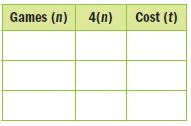• Type below: • Question 2 Tina drove 60 miles per hour to Tucson. Write a function rule that relates the number of miles traveled to the hours driven. • Type below: • Question 3 The Pizza Place is running a special for large pizzas. The cost of different numbers of pizzas is shown in the table. Write an equation to find the total cost t for a number of pizzas p.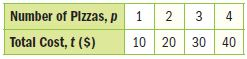• Type below: • Question 4 A baker used 16 ounces of butter for every batch of dough pretzels. Make a function table to show the relationship between the amount of butter b and the total batches of pretzels p. Write an equation to represent the situation.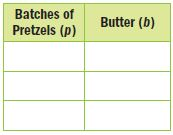• Type below: • Question 5 Valerie earns$25 a day plus $3 for each dog she washes. Make a function table to show the relationship between the number of dogs she washed d and the total amount earned t in one day. Write an equation to represent the situation.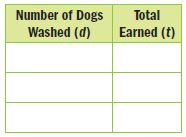• Type below: • Question 6 In order for an entire class to fit into a certain theater, there must be less than 34 students in the class. Use the inequality c < 34, where c is the class size to determine which classes fit in the theater.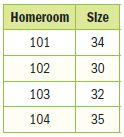• Type below: • Question 7 Reason Abstractly Vincent spends$4 to play a ring toss game at the fair. If he has at most $12, write and solve an inequality to find how many games of ring toss he can play. • Type below: Remodeling Project Mr. Jacobs is installing a new kitchen floor using white and brown tiles. The relationship between the number of brown tiles and the number of white tiles is shown in the table.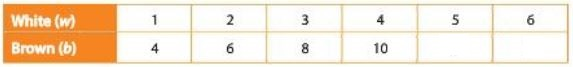Write your answers on another piece of paper. Show all of your work to receive full credit. • Question 8 Part A Fill in the missing values based on the pattern in the table. Write an equation that represents the relationship between the white tiles and the brown tiles. Let b represent brown tiles and w represent white tiles. • Type below: • Question 8 Part B Each tile costs$12. Determine the cost of 60 tiles, 80 tiles, 100 tiles, and 120 tiles. Write a set of ordered pairs (number of tiles, total cost) to represent the data.
Then graph the ordered pairs.

• Type below:
• Question 8

Part C

Mr. Jacobs budgeted $1,200 for tiles. His sketch of the floor requires him to use 38 white tiles. Each white tile costs$12. Write and solve an inequality to find the maximum amount he can spend on brown tiles.

• Type below:
• Question 8

Part D

The tile store has three different brown tiles Mr. Jacobs can use. The tile prices are shown in the table. Which tile(s) can he purchase to stay in his budget and meet the design for 38 white tiles? Explain your reasoning.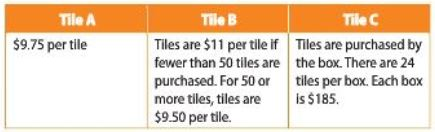• Type below:

Yes, email page to my online tutor. (if you didn't add a tutor yet, you can add one here)